# Determinate and Indeterminate Structures and Their DIfferencesReading time: 1 minute

Structure is generally classified into two categories as Determinate and Indeterminate Structures or Redundant Structures for analysis of structures to find forces based on criteria discussed below. Structure is an assemblage of a number of components like slabs, beams, columns, walls, foundations and so on, which remains in equilibrium. It has to satisfy the fundamental criteria of strength, stiffness, economy, durability and compatibility, for its existence. Any structure is designed for the stress resultants of bending moment, shear force, deflection, torsional stresses, and axial stresses. If these moments, shears and stresses are evaluated at various critical sections, then based on these, the proportioning can be done. Evaluation of these stresses, moments and forces and plotting them for that structural component is known as analysis. Determination of dimensions for these components of these stresses and proportioning is known as design.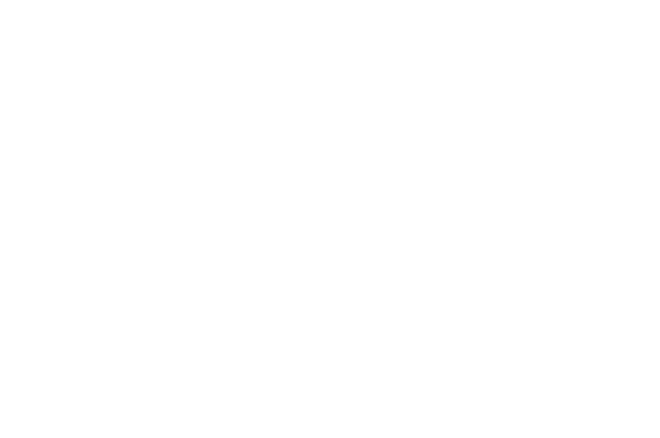Determinate structures are analysed just by the use of basic equilibrium equations. By this analysis, the unknown reactions are found for the further determination of stresses. Redundant or indeterminate structures are not capable of being analysed by mere use of basic equilibrium equations. Along with the basic equilibrium equations, some extra conditions are required to be used like compatibility conditions of deformations etc to get the unknown reactions for drawing bending moment and shear force diagrams. Example of determinate structures are: simply supported beams, cantilever beams, single and double overhanging beams, three hinged arches, etc. Examples of indeterminate structures are: fixed beams, continuous beams, fixed arches, two hinged arches, portals, multistoried frames, etc. Special methods like strain energy method, slope deflection method, moment distribution method, column analogy method, virtual work method, matrix methods, etc are used for the analysis of redundant structures.

## Indeterminate Structures

A structure is termed as statically indeterminate, if it can not be analysed from principles of statics alone, i.e.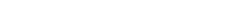. A statically indeterminate structure may be classified as:
1. Externally indeterminate, (example: continuous beams and frames shown in figure-1(a) and (b)).
2. Internally indeterminate, (example: trusses shown in figure-1(c) and (d)).
3. Both externally and internally indeterminate, (example: trussed beams, continuous trusses shown in figure-1 (e) and (f)).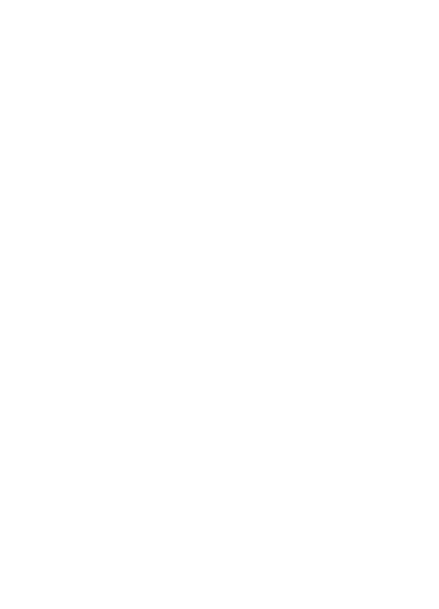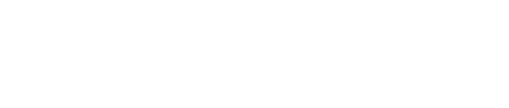Figure.1

### Externally Indeterminate Structures

A structure is usually externally indeterminate or redundant if the reactions at the supports can not be determined by using three equations of equilibrium, i.e.. In the case of beams subjected to vertical loads only, two reactions can be determined by conditions of equilibrium. Therefore, simply supported cantilever and overhanging beams shown in figure 2 are statically determinate structures.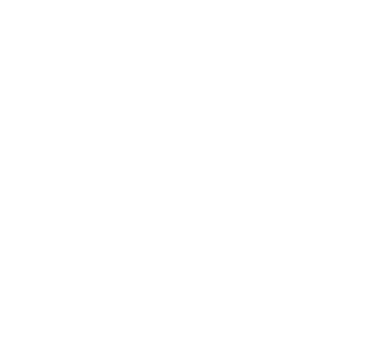Figure 2

If however a beam rests on more than two supports or in addition any of the end support is fixed, there are more than two reactions to be determined. These reactions can not be determined by conditions of equilibrium alone. The degree of indeterminacy or redundancy is given by the number of extra or redundant reactions to be determined. The beam shown in figure 3 (a) is statically indeterminate to one degree because there are three unknown reactions and statics has only two reactions. The beam in figure 3(b) is statically redundant to two degree. The beam in figure 3(c) is redundant to three degree and the beam in figure 3(d) is redundant to four degrees.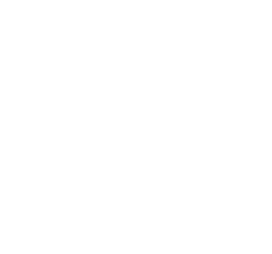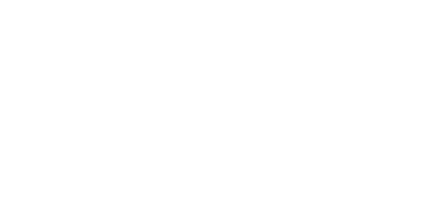Figure 3

A portal frame is statically determinate if there are only three external reactions, because there are three conditions of equilibrium for such a system. The portal frame shown in figure 4 are statically determinate because there are only three reactions to be determined. If a portal frame has more than three reactions it is statically indeterminate, the degree of indeterminacy or redundancy being equal to the number of redundant or extra reactions to be determined. Therefore, the portal frames of figure 5(a) and (b) are redundant by one degree, that of figure 5(c) is redundant by two degrees, that of figure 5(d) is redundant by three degrees, and that of figure 5(e) is redundant by 5 degrees.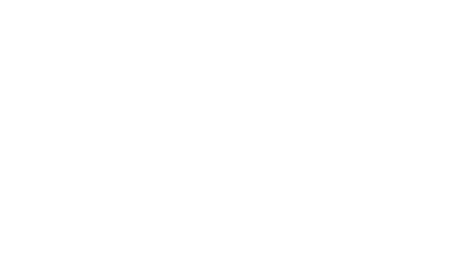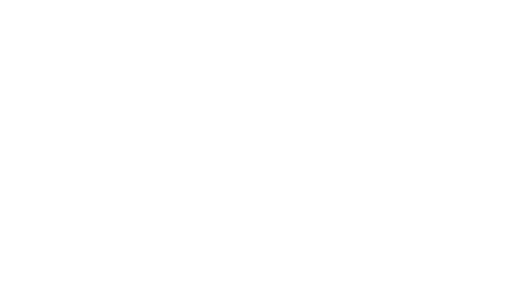Figure 4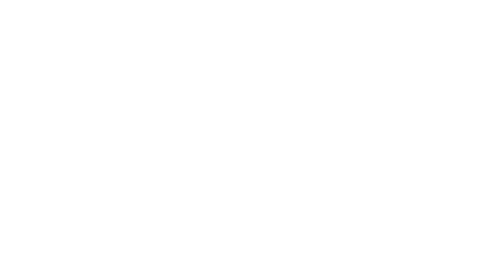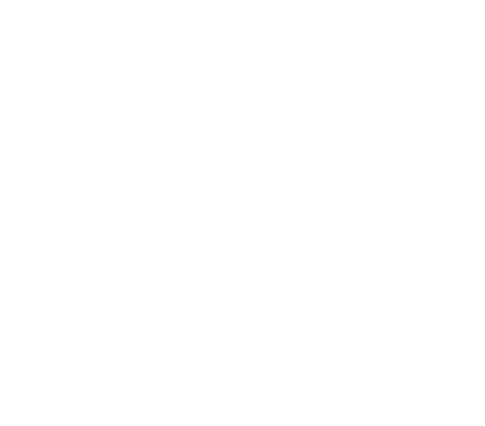Figure 5

The statically indeterminate beams and frames can be analysed by strain energy method, three moment equation, slope deflection method or moment distribution method.

### Internally Indeterminate Structures

A truss is statically determinate internally if the total number of members

m=2j – 3

where j= number of joints. A truss having more than (2j – 3) members is statically indeterminate or redundant, the degree of indeterminacy or redundancy being equal to the number of extra members.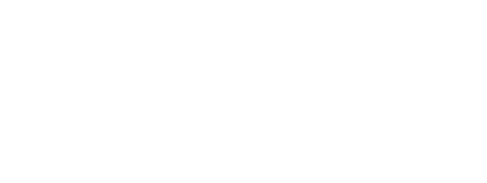Figure 6

Thus the truss shown in figure 6(a) is statically redundant by one degree because there are 14 members and 8 joints. Number of redundant members = m= 2j – 3 = 14 – (16 – 3) = 1 Similarly, the truss shown in figure 6(b) is internally redundant by two degrees. The internally indeterminate trusses can be analysed by strain energy method.

### Externally and Internally Indeterminate Structures

A truss is statically determinate, both externally and internally when (a) All the reactions can be determined from the conditions of equilibrium, namely, and (b) The total number of members, m= 2j – 3, where j= number of joints. The truss shown in figure 7 is externally indeterminate to one degree because the numbers of reactions to be determined are three, and the conditions of equilibrium reduces to two, namely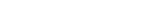. This truss is also internally indeterminate to one degree because there is one extra member. Number of redundant member = m – (2j – 3)= 22 – (2 x 12 – 3)= 1 Such trusses can be analysed by using strain energy method.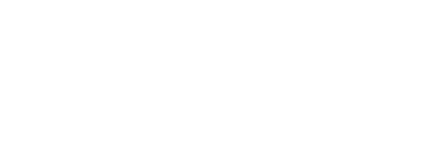Figure 7

## Difference Between Determinate and Indeterminate Structures

 S. No. Determinate Structures Indeterminate Structures 1 Equilibrium conditions are fully adequate to analyze the structure. Conditions of equilibrium are not adequate to fully analyze the structure. 2 Bending moment or shear force at any section is independent of the material property of the structure. Bending moment or shear force at any section depends upon the material property. 3 The bending moment or shear force at any section is independent of the cross-section or moment of inertia. The bending moment or shear force at any section depends upon the cross-section or moment of inertia. 4 Temperature variations do not cause stresses. Temperature variations cause stresses. 5 No stresses are caused due to lack of fit. Stresses are caused due to lack of fit. 6 Extra conditions like compatibility of displacements are not required to analyze the structure. Extra conditions like compatibility of displacements are required to analyze the structure along with the equilibrium equations.